# 12-240/Homework Assignment 2

• Note that the numbers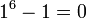$1^6-1=0$,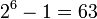$2^6-1=63$,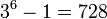$3^6-1=728$,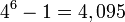$4^6-1=4,095$,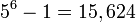$5^6-1=15,624$ and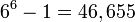$6^6-1=46,655$ are all divisible by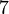$7$. The following four part exercise explains that this is not a coincidence. But first, let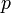$p$ be some odd prime number and let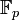${\mathbb F}_p$ be the field with p elements as defined in class.
1. Prove that the product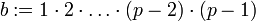$b:=1\cdot 2\cdot\ldots\cdot(p-2)\cdot(p-1)$ is a non-zero element of${\mathbb F}_p$.
2. Let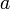$a$ be a non-zero element of${\mathbb F}_p$. Prove that the sets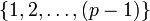$\{1,2,\ldots,(p-1)\}$ and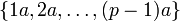$\{1a,2a,\ldots,(p-1)a\}$ are the same (though their elements may be listed here in a different order).
3. With$a$ and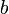$b$ as in the previous two parts, show that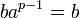$ba^{p-1}=b$ in${\mathbb F}_p$, and therefore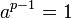$a^{p-1}=1$ in${\mathbb F}_p$.
4. How does this explain the fact that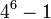$4^6-1$ is divisible by$7$?